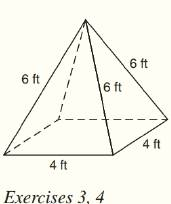Chapter 9.CT, Problem 4CTElementary Geometry For College St...

7th Edition
Alexander + 2 others
ISBN: 9781337614085

Solutions

Chapter
SectionElementary Geometry For College St...

7th Edition
Alexander + 2 others
ISBN: 9781337614085
Textbook Problem

For the regular square pyramid shown above, finda) the lateral area.b) the total area.To determine

a)

To find:

The lateral area of the regular square pyramid.

Explanation

Given:

The regular square pyramid is shown below

Formula used:

The lateral area of the regular pyramid is L=12lP where l is slant height and P is the perimeter of the base.

Calculation:

From the figure we see that the base has four edges each of 4 ft length.

Therefore the perimeter is 44=16 ft.

The triangular lateral face is an isosceles triangle as shown below

Here AD is the slant height l.

By Pythagoras theorem,

To determine

b)

To find:

The total area of the regular square pyramid.

Still sussing out bartleby?

Check out a sample textbook solution.

See a sample solution

The Solution to Your Study Problems

Bartleby provides explanations to thousands of textbook problems written by our experts, many with advanced degrees!

Get Started

let f(x) = x 1, g(x) = x+1, and h(x) = 2x3 1. Find the rule for each function. 15. fgh

Applied Calculus for the Managerial, Life, and Social Sciences: A Brief Approach

In Exercises 7-10, solve for x or y. (62)2+(2y)2=52

Calculus: An Applied Approach (MindTap Course List)

Find each sum 47+6.3+20.71+170.027

Elementary Technical Mathematics

Find f. f(t) = 3t 3/t, f(1) = 2, f(1) = 1

Single Variable Calculus: Early Transcendentals

Find the sum of odd integers between 14 and 58.

Finite Mathematics for the Managerial, Life, and Social Sciences